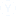what is the syllabus of PGT Mathematics
mathematics m.sc
• AV
• Anita
• 08 अक्तूबर
• 9848 दृश्य
• 2 उत्तर2 उत्तर
1-2 of  2
2 उत्तर
• Syllabus for PGT Mathematics Exam:
1. Sets
2. Relations & Functions
3. Principle of Mathematical Induction
4. Permutations & Combinations
5. Complex Numbers
6. Linear Inequalities
7. Sequence and Series
8. Elementary Number Theory
10. Matrices and Determinants
11. Two dimensional Geometry
12. Trigonometric Functions
13. Inverse Trigonometric Functions
14. Differential Calculus
15. Applications of Derivatives
16. Integral Calculus
17. Differential Equations
18. Vectors
19. Three dimensional Geometry
20. Statistics
21. Probability
22. Linear Algebra

• PGT (Mathematics) of  syllabus

Sets
Relations & Functions
Principle of Mathematical Induction
Permutations & Combinations
Complex Numbers
Linear Inequalities
Binomial Theorem
Sequence and Serie
Elementary Number Theory
Matrices and Determinant
Two dimensional Geometry
Trigonometric Functions
Inverse Trigonometric Functions
Differential Calculus
Applications of Derivatives
Integral Calculus
Differential Equation
Vector
Three dimensional Geometry
Statistics
Probability
Linear Algebraअन्य संबंधित चर्चाएंमॉक परीक्षण अभ्यास के लिए
Army Welfare Education Society (AWES) PGT TGT PRT